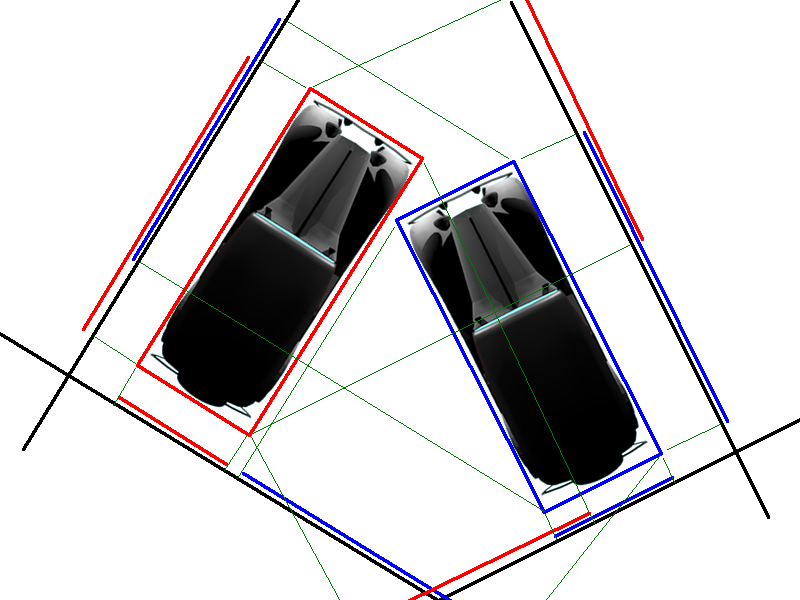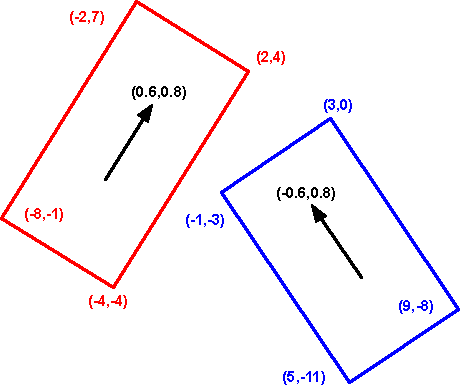# 3D Theory - Example - Car Racing Game - collisions

## Collision detection

### Collision between cars

Depending on how your game works, you may need more or less accurate collision detection. For instance in most cases the cars, on a given part of the track, will be traveling in the same direction therefore most shunts will be front to rear bumper. If we make this assumption we can use a very quick and easy method of collision detection such as bounding spheres (this is what we are using in the snooker example) This is fine for front to back collisions but if we drive into the side of a car the collision will be detected too early, I'm not sure this will be noticed when the cars are going fast, but in case you need it I will explain a more accurate method here.

Since the cars are almost rectangular when viewed from the top then, we can do collision detection by representing each car by its boundary rectangle.The problem then becomes: how do we detect when these rectangles overlap? Here is a good method (The mathematics behind it is explained on this page). If the axis of the rectangles were aligned it would be easy to check if they overlapped but since they are at different angles its a bit more tricky. If we were checking for a collision in the physical world we could walk around the objects, if we could see could see a gap between the objects from any direction, then the objects cant be overlapping.Since the objects are rectangular we only need to check along the planes of the rectangles which gives us four planes to check. We project the corner points of each rectangle onto each line. If the points of one rectangle is at one end of the line and the other rectangle is at the other end, with a gap in between, then the objects have not collided. We only need to find one line without an overlap to be sure there has not been a collision, only if all four lines have overlaps has a collision occurred.

In the diagram above, the line on the bottom left does not have an overlap, so the cars have not crashed.

Projecting onto a line is explained on this page. Since we only need to know how far along the vector the projected point is, all we need to do is take the dot product of the vector and the point, the result of this is a scalar quantity which is what we want.

To rotate the vector through 90 degrees we just swap the x and y coordinates and reverse one of them. (Thanks to TGA for reminding me about reversing one details here)

## Example

In this example we have two cars, the orientation of the red one is (0.6,0.8) and the orientation of the blue one is (-0.6,0.8).Lets first first project all the points onto the four possible vectors (here is the spreadsheet I used to calculate it collision.ods)

 x y dot red dot red 90 degrees dot blue dot blue 90 degrees red vector 0.6 0.8 overlap no overlap no overlap overlap blue vector -0.6 0.8 red corner 1 -2 7 4.4 -5.8 6.8 2.6 red corner 2 2 4 4.4 -0.8 2 4 red corner 3 -4 -4 -5.6 -0.8 -0.8 -5.6 red corner 4 -8 -1 -5.6 -5.8 4 -7 blue corner 1 3 0 1.8 2.4 -1.8 2.4 blue corner 2 9 -8 -1 12 -11.8 2.4 blue corner 3 5 -11 -5.8 10.6 -11.8 -2.6 blue corner 4 -1 -3 -3 1 -1.8 -2.6

As you can see the column marked:

• 'dot red' overlaps (red points cover range 1.8 to -5.8, some blue points in this range).
• 'dot red 90 degrees' does not overlap (red points are -ve, blue points are +ve).
• 'dot blue' does not overlap (red points are greater than -1, blue points are less than -1).
• 'dot blue 90 degrees' overlaps (red points cover range 2.6 to -5.6, blue points are in this range).

So the cars do not overlap as we only need to find one column which does not overlap to reject the possibility of a collision.

## Collision Response

What do we want to do if car hits obstacle or other car?

• game end ?
• change score ?
• change of direction?
• damage to car?
• explosion?